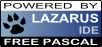## DataFrame and Simple Linear Regression

06 Aug 2017

In the previous post I mentioned using Iceberg successfully. The code I was pushing is SLRCalculator, a simple linear regression calculator, written to take Oleksandr Zaytsev's DataFrame library for a spin, by way of porting Jason Brownlee's excellent simple linear regression in Python to Pharo.

Firstly, install DataFrame. This also pulls in Roassal.

``````Metacello new
baseline: 'DataFrame';
repository: 'github://PolyMathOrg/DataFrame';
``````

SLRCalculator implements mean, variance, covariance, coefficients etc, and also incorporates the Swedish automobile insurance dataset used by Jason in his Python example.

``````SLRCalculator class>>loadSwedishAutoInsuranceData
"Source: https://www.math.muni.cz/~kolacek/docs/frvs/M7222/data/AutoInsurSweden.txt"
| df |

df := DataFrame fromRows: #(
( 108 392.5 )
( 19 46.2 )
( 13 15.7 )
"more lines" ).
df columnNames: #(X Y).
^ df
``````

The computation for covariance also uses DataFrame.

``````covariance: dataFrame
| xvalues yvalues xmean ymean covar |

xvalues := dataFrame columnAt: 1.
yvalues := dataFrame columnAt: 2.
xmean := self mean: xvalues.
ymean := self mean: yvalues.
covar := 0.
1 to: xvalues size do: [ :idx |
covar := covar + (((xvalues at: idx) - xmean) * ((yvalues at: idx) - ymean)) ].
^ covar
``````

Let's see how to use SLRCalculator to perform linear regression, with graphing using Roassal. First declare the variables and instantiate some objects:

``````| allData splitArray trainingData testData s coeff g dsa dlr legend |

s := SLRCalculator new.

``````

Next, split the data set into training and test subsets. Splitting without shuffling means to always take the first 60% of the data for training.

``````splitArray := s extractForTesting: allData by: 60 percent shuffled: false.
trainingData := splitArray at: 1.
testData := splitArray at: 2.
coeff := s coefficients: trainingData.
``````

Set up for graphing. Load `allData' as points.

``````g := RTGrapher new.

allData do: [ :row |
dsa := RTData new.
dsa dotShape color: Color blue.
dsa points: { (row at: 1) @ (row at: 2) }.
dsa x: #x.
dsa y: #y.
``````

Create the points to plot the linear regression of the full data set, using the coefficients computed from the training subset.

``````dlr := RTData new.
dlr noDot.
dlr connectColor: Color red.
dlr points: (allData column: #X).
" y = b0 + (b1 * x) "
dlr x: #yourself.
dlr y: [ :v | (coeff at: 1) + (v * (coeff at: 2)) ].
``````

Make the plot look nice.

``````g axisX noDecimal; title: 'Claims'.
g axisY title: 'Total payment in SEK'.
g shouldUseNiceLabels: true.
g build.

legend := RTLegendBuilder new.
legend view: g view.
legend addText: 'Swedish Automobile Insurance Data Linear Regression'.
legend build.

g view
``````

Putting the code altogether:

``````| allData splitArray trainingData testData s coeff g dsa dlr legend |

s := SLRCalculator new.

splitArray := s extractForTesting: allData by: 60 percent shuffled: false.
trainingData := splitArray at: 1.
testData := splitArray at: 2.
coeff := s coefficients: trainingData.

g := RTGrapher new.

allData do: [ :row |
dsa := RTData new.
dsa dotShape color: Color blue.
dsa points: { (row at: 1) @ (row at: 2) }.
dsa x: #x.
dsa y: #y.

dlr := RTData new.
dlr noDot.
dlr connectColor: Color red.
dlr points: (allData column: #X).
" y = b0 + (b1 * x) "
dlr x: #yourself.
dlr y: [ :v | (coeff at: 1) + (v * (coeff at: 2)) ].

g axisX noDecimal; title: 'Claims'.
g axisY title: 'Total payment in SEK'.
g shouldUseNiceLabels: true.
g build.

legend := RTLegendBuilder new.
legend view: g view.
legend addText: 'Swedish Automobile Insurance Data Linear Regression'.
legend build.

g view
``````

Copy/paste the code into a playground, press shift-ctrl-g...Tags: data science, machine learning